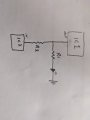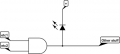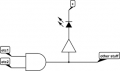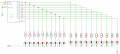# Understanding voltage dividers on ic output

Joined Mar 4, 2020
41
Hi everyone,
In the following circuit:Ic1 has an output pin that sends a signal to ic2 and powers the LED.

How do I know if R1 would create a voltage divider? If there is a resistor in ic1 (let's call it Ric), then a divider would be formed by R1 and Ric, and then ic2 would only receive a fraction of the expected voltage.

Is this never a problem? Or if it is, is there something in the datasheet of ic1 that would indicate the value of Ric? In my case, I'm using logic gates. But my question is applicable to any other ic like uC

Thank you.

#### ericgibbs

Joined Jan 29, 2010
17,446
hi yp,
Which type of logic gate are you using,?
E

Joined Mar 4, 2020
41
I'm using a binary counter as ic1 and a Not gate as ic2. But my question is more general to all ic since I can think of many other examples where the same question applies. For example, ic1 would be a uC, ic2 would be a opamp.

Am I right to assume to this could potentially create a divider? Or is this never a problem?

#### ericgibbs

Joined Jan 29, 2010
17,446
hi yp,
I would say any load on a logic output pin will cause the Vout level to fall from its off load state.
Usually, any additional load should be chosen so that the following gate input receives what it would consider a logic High.
E

Joined Mar 4, 2020
41
Thank you @ericgibbs . So in the case where the ic2 is an analog component, like an opamp or and ADC, the vout is more critical since it might be underible to drop as few as 0.2v. does this mean that an LED should never be placed in the circuit as shown above?

I'd like to understand why there is a voltage drop. If I replace ic1 by a 5v source, I can measure 5v on the input of ic2. But with ic1 in place, I currently measure 3.5v on the input of ic2 when uring a red rgb with R1=330.

With the 5v source experiment, if I put a resistor right at the begining of the circuit, then that resistor, with R1, creates a voltage divider. I am concluding that an ic is like a voltage source with a resistance.

If that's the case, Should I be able to find that resistance value in the datasheet (cd4017 in my case) so that I can try to adjust the circuit. Maybe by connecting the led thru a transistor instead, but without knowing the ic's output resistance, I can't properly calculate the gate resistor value.

#### BobTPH

Joined Jun 5, 2013
6,528
1. Normally we would not put a resistor between an output and an input. R2 is generally not needed, especially if the out and in are of the same logic family.

2. Any modern CMOS input draws so little current, it would have little effect on the output voltage.

Bob

#### Alec_t

Joined Sep 17, 2013
13,446
The output impedance of IC1 is important. If it is high (more than a few Ohms), then the load presented by R1 and the LED will pull down the voltage at the IC1 output pin significantly.

Joined Mar 4, 2020
41
@ericgibbs . I'm not sure how that indicates what the output impedence is. Although I feel like this is related to V=RI. I'd appreciate it if you could explain how this value (from the datasheet) in combination with the load at R2 will impact the voltage at ic2.

Thank you

#### MrChips

Joined Oct 2, 2009
28,530
When you drive an LED directly (via a series resistor) from an IC output you disturb the voltage output from the IC.

You should never drive an LED and another IC from the one logic output.

Don't do this:In other words, use a separate buffer/driver for the LED.

Joined Mar 4, 2020
41
Can someone validate if I understand this right: If 20ma is the max current for an output pin and voltage is 5v, then it means that the pin impedence is 5/0.02=250 ohms.
so by having a load of 330ohm on R2 (as per my first post's drawing), I am effectively creating a voltage divider with R1=250, R2=330. So ic2 would then only get 2.84v

Is that accurate? If yes, then I understand why this is happening

EDIT: So knowing that output impedence of ic1 is 250ohm, then I can calculate that if I power the LED thru a transistor with a base connected on a 20k resistor,ic2 would only see a voltage drop of 0.1v

Last edited:

#### MrChips

Joined Oct 2, 2009
28,530
Your calculation of 5V/0.02A is not correct for measuring the output resistance.
What you need to measure is the loss in voltage from no load to loaded.

Why not just take a voltmeter and measure the voltage at the receiving IC under logic LOW and HIGH conditions in your test circuits? Then decide if the voltages meet the input logic thresholds with adequate margin for error.

#### Ian0

Joined Aug 7, 2020
7,484
You can see from the table in post #4 that for a supply of 5V, for a typical device, and at at temperature of 25°C the output is 4.6V at an output of 1mA.
So the output has lost 0.4V from the 5V supply.
The output resistance is therefore 0.4V/1mA = 400Ω
If the load is increased to 3.2mA, (next line down in the table) the voltage loss is now 2.5V - the resistance is now 780Ω - it is heading towards a constant current mode.
You can calculate the resistance, but bear In mind that it is not linear, it varies with supply voltage and temperature, and there is a variation of more than 2:1 between devices, but it is still useful to know an approximate value.
HC logic is much better the 4000 CMOS with output resistances around 50Ω.

•Joined Mar 4, 2020
41
Thank you @Ian0 . I understand clearly now.
So what surprises me is the following design is very popular:I was trying to reproduce it but couldn't make it work in a way that it would be possible to get the full 5V out of the CV output. So it seems that even though it is very popular, it might be incorrect. I'm gonna build this with buffers after each outputs of the 4017.

#### Audioguru again

Joined Oct 21, 2019
5,823
The Texas Instruments datasheets for CD4xxx Cmos devices show a maximum allowed output of 100mW for each output.
They show a graph of typical amount of output current and output voltage at 5V, 10V and 15V supply voltages.
The typical output current with a 5V supply and a 2.5V LED without a current-limiting resistor is only 3.5mA. Then the output transistor heats with 2.5V x 3.5mA= 8.75mW which is fine.

With a 10V supply, the typical output into a 2.5V LED without a current-limiting resistor is 18mA. Then the output transistor has 10V - 2.5V= 7.5V across it and it heats with 7.5V x 18mA= 135mW which is much too high and it will be destroyed.

The output voltage is the supply voltage when the load current is extremely low. The graphs on the datasheet show curves with the three supply voltages and the typical output voltage with various load currents.

•LowQCab